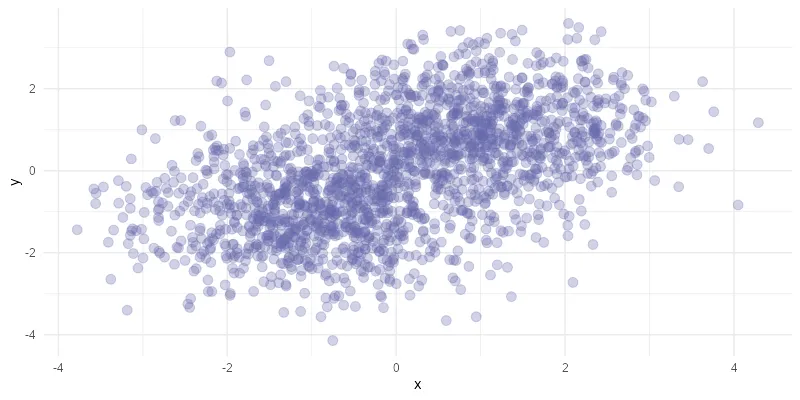# Convert images to WebP in R programming

It is possible to export or convert images to WebP format by using R programming. Images in WebP format mostly have much smaller sizes than in the JPEG or PNG format. If you are using images on websites, this format is worth considering.

The key element to converting images to WebP format in R is the WebP package that is compatible with the jpeg and png packages.

## Export image in WebP format in R

Here is my R plot that I would like to save in WebP format.

```require(ggplot2)

set.seed(12345)

x <- c(rnorm(1000, mean = -1), rnorm(1000, mean = 1))
y <- c(rnorm(1000, mean = -1), rnorm(1000, mean = 1))

xy <- data.frame(x, y)

ggplot(data = xy, aes(x = x, y = y)) +
geom_point(color = "#6667AB",
size = 3,
alpha = 0.3) +
theme_minimal()```One of the ways is to save the R plot temporarily in a PNG file un use that to convert it into WebP.

```# save the R plot temporarily

tp_path <- tempfile("myplot_", fileext = ".png")

ggsave(
tp_path,
width = 800,
height = 400,
units = "px",
dpi = 100,
bg = "white"
)

# convert temporary plot into WebP

require(webp)
require(png)

```

You can control the quality of the outcome with the corresponding argument in the function write_webp.

## Convert multiple images to WebP in R

You can use this method in similar file-type conversion situations. Here is an example if you want to convert multiple PNG images to WebP format in R. It is done using the package purrr and is a similar loop that is used to combine various Excel files.

First of all, let’s create a few R plots in PNG format.

```require(ggplot2)

set.seed(12345)

x <- c(rnorm(1000, mean = -1), rnorm(1000, mean = 1))
y <- c(rnorm(1000, mean = -1), rnorm(1000, mean = 1))

xy <- data.frame(x, y)

p1 <- ggplot(data = xy, aes(x = x, y = y)) +
geom_point(color = "#6667AB",
size = 3,
alpha = 0.3) +
theme_minimal()

ggsave(
plot = p1,
width = 800,
height = 400,
units = "px",
dpi = 100,
bg = "white"
)

ggsave(
plot = p1,
width = 800,
height = 400,
units = "px",
dpi = 100,
bg = "white"
)```

The next step is to get file paths for all the necessary files and replace the current file extension with WebP in it. That is necessary for the purrr loop to convert all the images.

```require(purrr)
require(webp)
require(png)

l1 <- list.files(
path = path,
pattern = "*.png",
full.names = TRUE
)

l2 <- gsub('png', 'webp', l1)

walk2(l1, l2, ~ write_webp(readPNG(.x), target = .y))```

If you want to create a progress bar for this process, look at this post. Usually, in the purrr loops, the function map2 is used instead of walk2. In this situation, I don’t want the additional console output that comes from the map2 function.

## Convert JPEG to WebP in R

This is a little extra for situations when you have to deal with JPEG format. Converting from JPEG to WebP is similar to the example with PNG. It is necessary to have the jpeg package.

```require(ggplot2)

set.seed(12345)

x <- c(rnorm(1000, mean = -1), rnorm(1000, mean = 1))
y <- c(rnorm(1000, mean = -1), rnorm(1000, mean = 1))

xy <- data.frame(x, y)

ggplot(data = xy, aes(x = x, y = y)) +
geom_point(color = "#6667AB",
size = 3,
alpha = 0.3) +
theme_minimal()

# save the R plot temporarily

tp_path <- tempfile("myplot_", fileext = ".jpg")

ggsave(
tp_path,
width = 800,
height = 400,
units = "px",
dpi = 100,
bg = "white"
)

# convert temporary plot into WebP

require(webp)
require(jpeg)